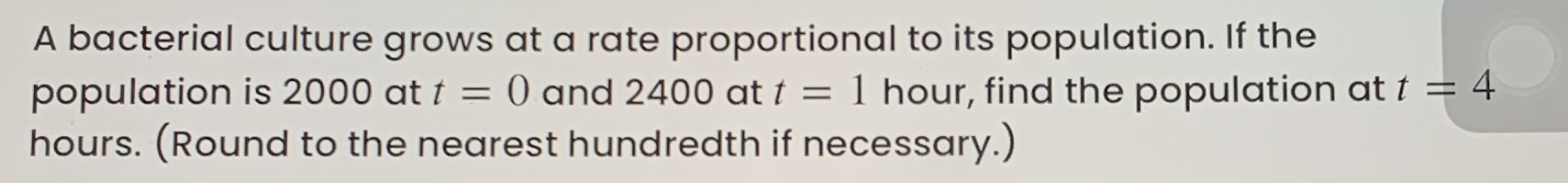### ¿Todavía tienes preguntas de matemáticas?

Pregunte a nuestros tutores expertos
Algebra
PreguntaA bacterial culture grows at a rate proportional to its population. If the population is $$2000$$ at $$t = 0$$ and $$2400$$ at $$t = 1$$ hour, find the population at $$t = 4$$ hours. (Round to the nearest hundredth if necessary.)